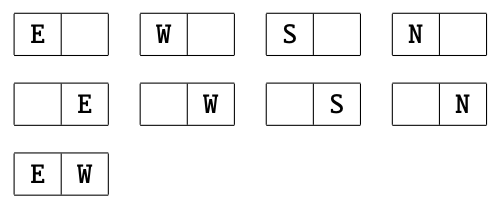시간 제한 메모리 제한 제출 정답 맞은 사람 정답 비율
2 초 512 MB 16 16 15 100.000%

## 문제

JOI-kun runs a campsite. This campsite is divided into a rectangular grid of H rows and W columns. The rows are parallel to the east-west direction, and the columns are parallel to the north-south direction. The section in the i-th row from north and j-th column from east is called the section (i, j).

JOI-kun is going to put up some tents in some sections. Each tent must occupy exactly one section. No two tents may occupy the same section.

Each tent has one entrance directed to one of the four directions: north, south, east or west. The directions of the entrances of the tents put up in the campsite must satisfy the following conditions.

• If both of the two sections (i1, j) and (i2, j) (1 ≤ i1 < i2 ≤ H, 1 ≤ j ≤ W) are occupied by tents, then the entrance of the tent in the section (i1, j) must be directed to south, and the entrance of the tent in the section (i2, j) must be directed to north.
• If both of the two sections (i, j1) and (i, j2) (1 ≤ i1 < i2 ≤ H, 1 ≤ j ≤ W) are occupied by tents, then the entrance of the tent in the section (i, j1) must be directed to east, and the entrance of the tent in the section (i, j2) must be directed to west.

JOI-kun became curious about the number of ways to put up at least one tent in the campsite. Two ways to put up tents are distinguished when there exists a section such that the status of the tent in the section (existence of a tent, or the direction of the entrance of the tent) differs.

Write a program which calculates the remainder of the number of ways to put up at least one tent satisfying the condition described in the problem statement, divided by 1 000 000 007.

## 입력

Read the following data from the standard input.

• The first line of input contains two integers H and W. This means that the campsite ran by JOI-kun is divided into H rows and W columns.

## 출력

Write one line to the standard output. The output should contain the remainder of the number of ways to put up at least one tent satisfying the condition described in the problem statement, divided by 1 000 000 007.

## 제한

• 1 ≤ H ≤ 3 000.
• 1 ≤ W ≤ 3 000.

## 예제 입력 1

1 2


## 예제 출력 1

9


Let us denote a tent with the entrance directed to east, west, south and north by the characters ’E’, ’W’, ’S’, ’N’, respectively. There are nine ways to put up some tents as illustrated below.## 예제 입력 2

4 3


## 예제 출력 2

3252


## 예제 입력 3

100 100


## 예제 출력 3

561068619